Test: The Laplace Transform

# Test: The Laplace Transform

Test Description

## 20 Questions MCQ Test | Test: The Laplace Transform

Test: The Laplace Transform for Railways 2023 is part of Railways preparation. The Test: The Laplace Transform questions and answers have been prepared according to the Railways exam syllabus.The Test: The Laplace Transform MCQs are made for Railways 2023 Exam. Find important definitions, questions, notes, meanings, examples, exercises, MCQs and online tests for Test: The Laplace Transform below.
Solutions of Test: The Laplace Transform questions in English are available as part of our course for Railways & Test: The Laplace Transform solutions in Hindi for Railways course. Download more important topics, notes, lectures and mock test series for Railways Exam by signing up for free. Attempt Test: The Laplace Transform | 20 questions in 60 minutes | Mock test for Railways preparation | Free important questions MCQ to study for Railways Exam | Download free PDF with solutions
 1 Crore+ students have signed up on EduRev. Have you?
Test: The Laplace Transform - Question 1

### Given the transform pair below. Determine the time signal y(t) and choose correct option.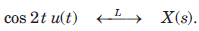​ ​ ​ Que: Y(s) = (s + 1)X(s)

Detailed Solution for Test: The Laplace Transform - Question 1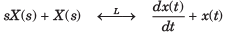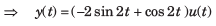Test: The Laplace Transform - Question 2

### Given the transform pair below. Determine the time signal y(t) and choose correct option.Que: Y(s) = X(3s)

Detailed Solution for Test: The Laplace Transform - Question 2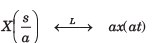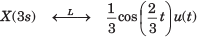Test: The Laplace Transform - Question 3

### Given the transform pair below. Determine the time signal y(t) and choose correct option.Que: Y(s) = X(s + 2)

Detailed Solution for Test: The Laplace Transform - Question 3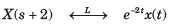Test: The Laplace Transform - Question 4

Unilateral Laplace Transform is applicable for the determination of linear constant coefficient differential equations with ________

Test: The Laplace Transform - Question 5

Given the transform pair below. Determine the time signal y(t) and choose correct option.Que: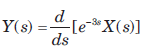Detailed Solution for Test: The Laplace Transform - Question 5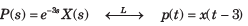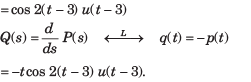Test: The Laplace Transform - Question 6

Given the transform pair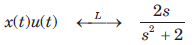Determine the Laplace transform Y(s) of the given time signal in question and choose correct option.

Que: y(t) = x(t - 2)

Detailed Solution for Test: The Laplace Transform - Question 6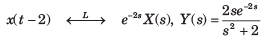Test: The Laplace Transform - Question 7

Given the transform pairDetermine the Laplace transform Y(s) of the given time signal in question and choose correct option.

Que: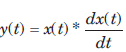Detailed Solution for Test: The Laplace Transform - Question 7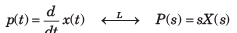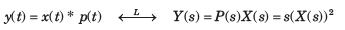Test: The Laplace Transform - Question 8

Given the transform pairDetermine the Laplace transform Y(s) of the given time signal in question and choose correct option.

Que: y(t) = e-t x(t)

Detailed Solution for Test: The Laplace Transform - Question 8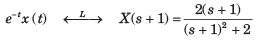Test: The Laplace Transform - Question 9

Given the transform pairDetermine the Laplace transform Y(s) of the given time signal in question and choose correct option.

Que: y(t) = 2tx(t)

Detailed Solution for Test: The Laplace Transform - Question 9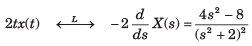Test: The Laplace Transform - Question 10

Determine the bilateral laplace transform and choose correct option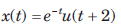​ ​ ​

Detailed Solution for Test: The Laplace Transform - Question 10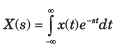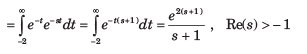Test: The Laplace Transform - Question 11

Determine the bilateral laplace transform and choose correct option.

x(t) = u(-t + 3)

Detailed Solution for Test: The Laplace Transform - Question 11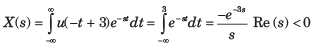Test: The Laplace Transform - Question 12

Determine the bilateral laplace transform and choose correct option

y(t) = δ(t + 1)

Detailed Solution for Test: The Laplace Transform - Question 12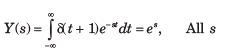Test: The Laplace Transform - Question 13

Determine the bilateral laplace transform and choose correct option

x(t) = sin t u(t)

Detailed Solution for Test: The Laplace Transform - Question 13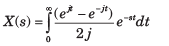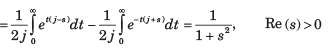Test: The Laplace Transform - Question 14

Determine the bilateral laplace transform and choose correct option.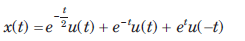Detailed Solution for Test: The Laplace Transform - Question 14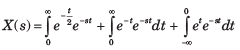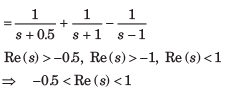Test: The Laplace Transform - Question 15

Determine the bilateral laplace transform and choose correct option.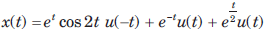Detailed Solution for Test: The Laplace Transform - Question 15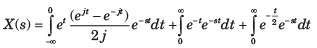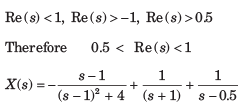Test: The Laplace Transform - Question 16

Determine the bilateral laplace transform and choose correct option.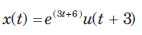​ ​ ​

Detailed Solution for Test: The Laplace Transform - Question 16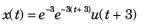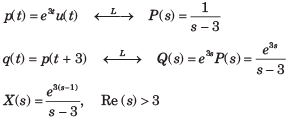Test: The Laplace Transform - Question 17

Where is the ROC defined or specified for the signals containing causal as well as anti-causal terms?

Test: The Laplace Transform - Question 18

The transform function H(s) of a causal system is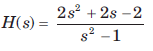The impulse response is

Detailed Solution for Test: The Laplace Transform - Question 18

For a causal system h(t) = 0 for t < 0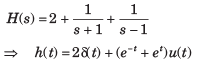Test: The Laplace Transform - Question 19

The transfer function H(s) of a stable system is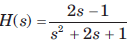The impulse response is

Detailed Solution for Test: The Laplace Transform - Question 19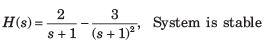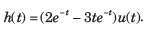Test: The Laplace Transform - Question 20

The transfer function H(s) of a stable system is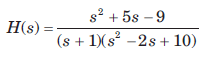The impulse response is

Detailed Solution for Test: The Laplace Transform - Question 20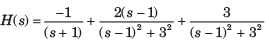System is stable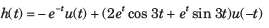Information about Test: The Laplace Transform Page
In this test you can find the Exam questions for Test: The Laplace Transform solved & explained in the simplest way possible. Besides giving Questions and answers for Test: The Laplace Transform, EduRev gives you an ample number of Online tests for practice(Scan QR code)